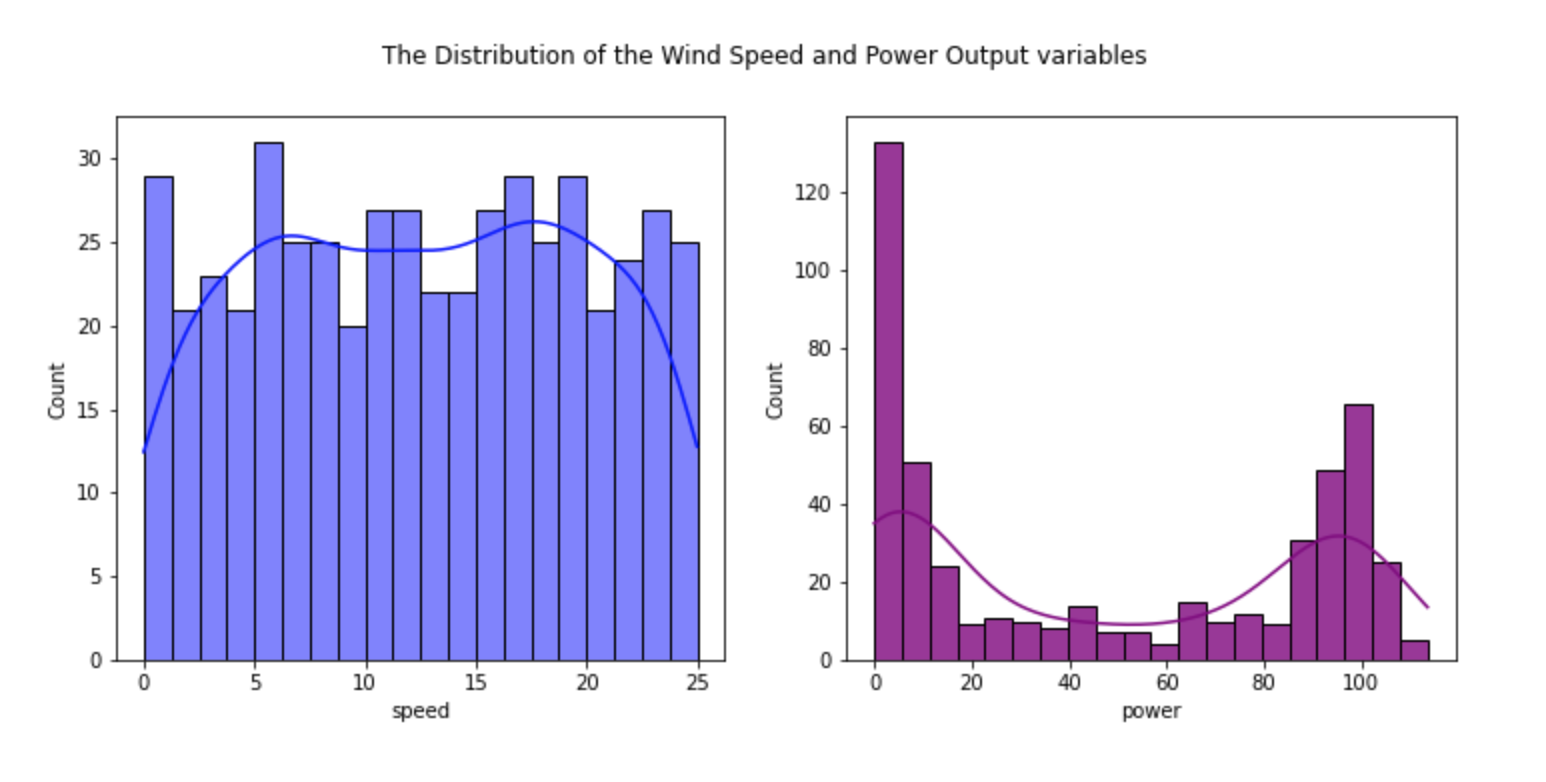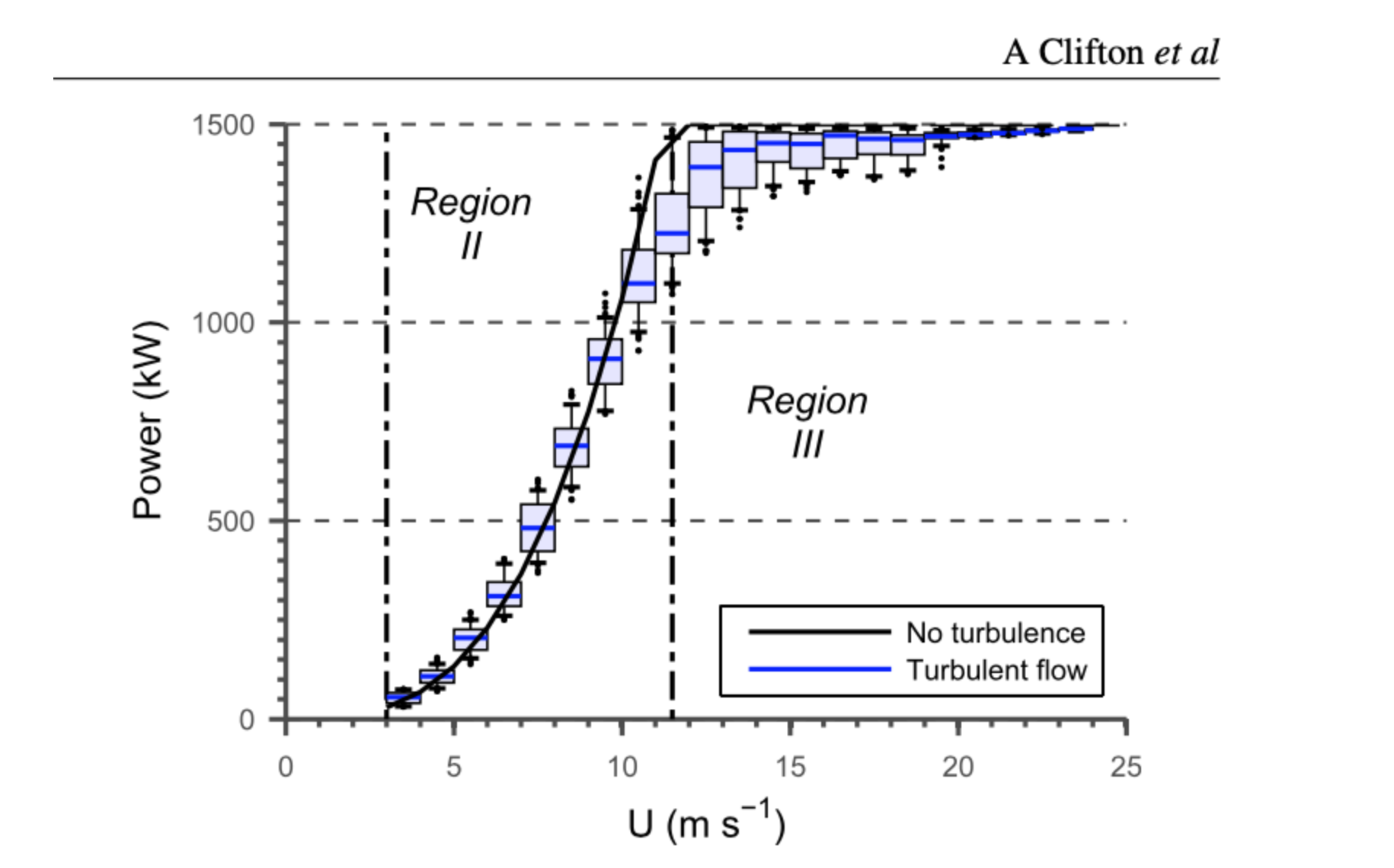s

## Machine Learning and Statistics Project

#### Predicting power output from wind turbines based on wind speed valuesMachine-learning project: Predicting power output from wind speed.

This is an overview of the project I completed for the Machine Learning and Statistics module of the Higher Diploma in Computing and Data Analytics at GMIT.

#### Description:

The aim of the project was to create a web service that used machine learning to make predictions for power output from wind turbines based on their wind speed values. The dataset consisted of 500 observations of wind speed and power output values with the following distributions in Figure 1 below.Figure 1: The distribution of Wind Speed and Power Output variables in the dataset

Figure 2 shows the s-shaped power curve as illustrated in a study by A Clifton, L Kilcher, J K Lundquist and P Fleming on using machine learning to predict wind turbine power output.Figure 2: Using machine learning to predict wind turbine_power output by A Clifton, L Kilcher, J K Lundquist and P Fleming, ENVIRONMENTAL RESEARCH LETTERS, doi:10.1088/1748-9326/8/2/024009, Environ. Res. Lett. 8 (2013) 024009 (8pp) at https://www.researchgate.net

The project was developed in a Jupyter notebook in which I trained some models using the data set, explain the models and give an analysis of their accuracy. The full project is available at GitHub.com.

The notebook is also available to read here.

I used both Polynomial Regression models and Artificial Neural Networks (Multiple Perceptron Layer) to make predictions of the expected power output given a particular wind speed value.

Here is a plot showing how the polynomial regression models compared to the artificial neural network models.

#### The Web application

• The project also involved writing a Flask web application program that allows a user to enter a wind speed value and in return outputs a prediction for the wind turbine power output based on that wind speed. See application.py for the Python code for creating the web application.

This section contains the following sections:

• Python packages used in the project
• Importing the required Python modules
• Running the web application

### Exploratory Data Analysis on the dataset

This section contains the following sections:

• The distribution of the data
• Some summary statistics
• Regression plots

### Some background on Wind Turbines power output

This section contains the following sections: Some research on wind turbines including:

• How wind speed and power output are measured
• The three main characteristic speeds of a typical wind turbine

### A closer look at the data

This section contains the following sections:

• Looking at the data in more detail
• Zero and non-zero values; dealing with missing values
• Some data cleaning

### Machine Learning Model 1 - Polynomial Regression

This section contains the following sections:

• Some background on regression and why it might be a suitable model to use
• Splitting the data into training and test sets
• Transforming features
• Fitting the model to the training data
• Predicting using the polynomial regression model
• Visualising the Polynomial regression results
• Evaluating the model
• Saving the polynomial regression models for use in the web application

### Machine Learning model 2 - Artificial Neural Networks

This section contains the following sections:

• Some background on an artificial neural networks model and why it might be a suitable model to use
• Splitting the data into training and test sets
• Defining the model
• Compiling the model
• Fitting the model to the training data
• Evaluating the model
• Plotting the learning curves
• Evaluating the performance of the model on new data
• Predicting using the artifical neural network model
• Comparing the actual data to predictions on the test set
• Saving the model for use in the web application

### Comparing the models

This section contains the following sections:

### Summary and conclusions

This section contains some summary notes on the project and conclusions.

### References

This section contains a list of all the references used in researching and developing the project.##### Tech used:
• Python
• Jupyter Notebook
• Scikit-learn
• Keras Tensorflow
• Pandas
• NumPy
• SciPy
• Matplotlib
• Seaborn